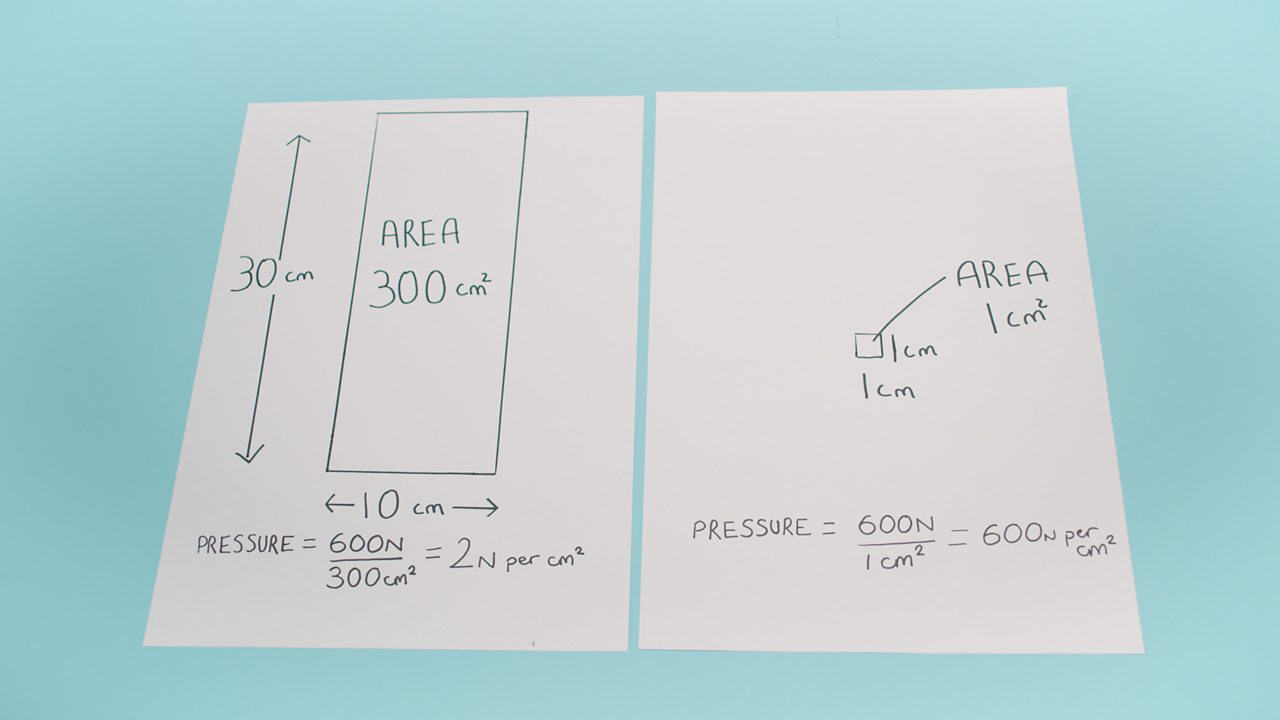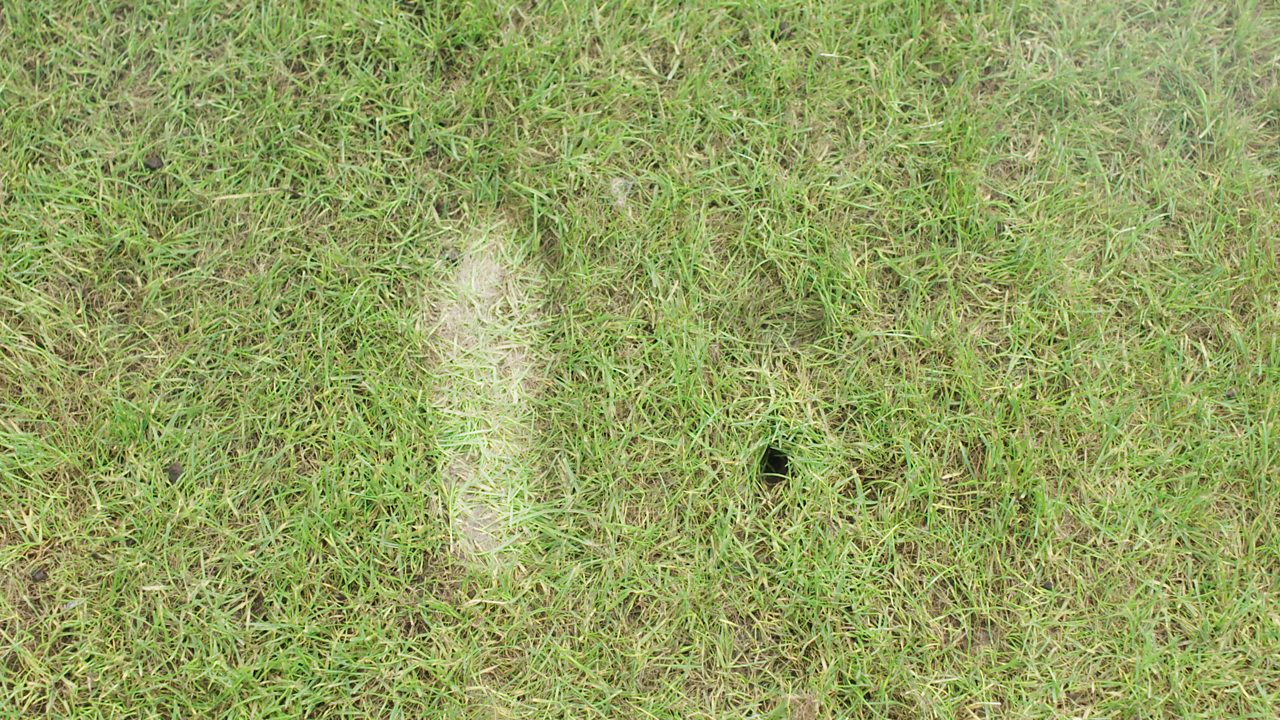How to show the difference between force and pressure

• A force can be a push or a pull. When a force is exerted on an object it can change the object's speed, direction of movement or shape.

• Pressure is a measure of how much force is acting upon an area.

• Pressure can be found using the equation pressure = force / area. Therefore, a force acting over a smaller area will create more pressure.

Have a goClick to see a step-by-step slideshow.1 of 7YOU WILL NEED: Weighing scales, calculator, ruler, pen, trainer, stiletto shoe and a piece of paper.2 of 7STEP 1: Use the scales to find your mass in kilograms.3 of 7STEP 2: Stand on a sheet of paper and use a pen and a ruler to draw a rectangle around the trainer. Do the same with the stiletto heel.4 of 7STEP 3: Measure the sides of each rectangle and calculate their area. Finally calculate pressure.5 of 7STEP 4: Put on the stiletto and stand on the grass. Do the same with the trainer.6 of 7STEP 5: Observe the difference in pressure, by looking at the two footprints produced. The stiletto creates the biggest pressure, so it has made a hole.7 of 7

How do we measure pressure?

Mass is a measure of how much matter there is; force is the pull of the Earth's gravity on that mass. Each kg of mass is pulled down by the Earth with a force of 10 N.

We all have a mass (kg). To convert this to force (N) we multiply the mass by 10.

We can then calculate pressure using: pressure = force ÷ area.

If you stand on one foot (rather than both feet), the pressure exerted would be twice as great. Similarly, a stiletto heel creates far more pressure than a normal shoe.

Did you know?

A person wearing a stiletto can exert more pressure on the ground than an army tank! This is because the army tank uses caterpillar tracks to spread its weight (and therefore force) over a larger area, in order to reduce the pressure. If it didn't, it would sink into the ground and wouldn't get very far!

Who uses knowledge of forces and pressure?

When structural engineers and architects design and construct a building, they need to make sure the foundation is strong enough and that the building doesn't sink into the ground.